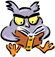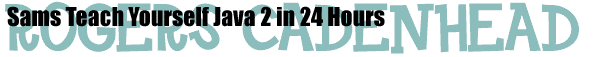## Hour 8: Repeating an Action With Loops

#### NOTES AND CORRECTIONS

• On Page 132, the second exercise is a poor choice on my part because it requires techniques that haven't been introduced in the first eight hours of the book.
Here's a more suitable assignment: Write a short program using loops that finds the first 400 numbers that are multiples of 13. The solution for this exercise can be found in Find13s.java and Find13s.class.

#### SOURCE FILES

• Solution #2: FindPrimes.java and Find400Primes.class. To find out if a number is prime, you have to use the``` %``` operator in Java, which returns the remainder of a division operation. If you divide a number `x` by every number from 2 through the square root of `x`, any division that has a 0 remainder means that `x` is not prime. This can be used to find the first 400 prime numbers. for more information on primes, visit the Prime Page.